# High School Chemistry : Calculating Solubility

## Example Questions

### Example Question #1 : Calculating Solubility

Barium fluoride is an insoluble salt.

If the solubility product constant of barium fluoride is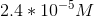, what is the solubility of barium fluoride?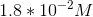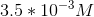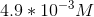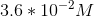Explanation:

If given the solubility product constant for a salt, we can determine the solubility for the salt as well. In order to do this, we need to use an ICE table and the equilibrium constant expression.

Initial: When the salt is added to water, there are no ions initially in solution. Because the salt is a solid, its concentration is irrelevant.

Change: When one molecule of barium fluoride dissolves, one barium ion and two fluoride ions are introduced to the solution. As a result, barium ions increase by, and fluoride ions increase by.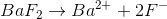End: The equilibrium expression for this salt is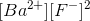, which will allow us to calculate the solubility based on the change in ion concentration.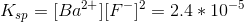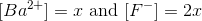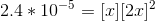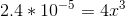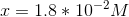The solubility for barium fluoride is.

### Example Question #1 : Calculating Solubility

For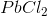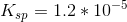Determine the maximum amount of grams ofthat will dissolve in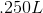of water at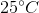.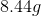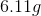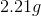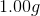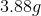Explanation:

Definition of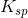: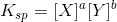for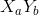.

For: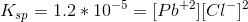Due to the chemical formula, there will be twice as many chloride ions as lead ions.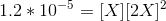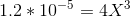Solve for the unknown variable: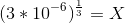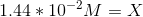Multiply times the given volume: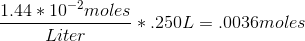Multiply times the molar mass of: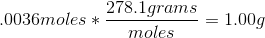### All High School Chemistry Resources